@Team 2019-06-09T15:53:00.000000Z 字数 4291 阅读 2930

# 图像超分辨率网络：EDSR

## 2、存在问题

（1）、神经网络模型的重建性能对架构的微小变化很敏感。同样的模型在不同的初始化和训练技术之下实现的性能水平不同。
（2）、大多数现有的SR算法将不同缩放因子的超分辨率问题作为独立的问题，没有考虑并利用SR中不同缩放之间的相互关系。 因此，这些算法需要许多scale-specific的网络，需要各自进行训练来处理各种scale。
（3）、SRResNet 成功地解决了计算时间和内存的问题，并且有很好的性能，但它只是采用了原始的ResNet架构，而原始的ResNet目的是解决更高层次的计算机视觉问题，例如图像分类和检测。因此，将ResNet架构直接应用于超分辨率这类低级视觉问题可能不是最佳的。

## 3、方法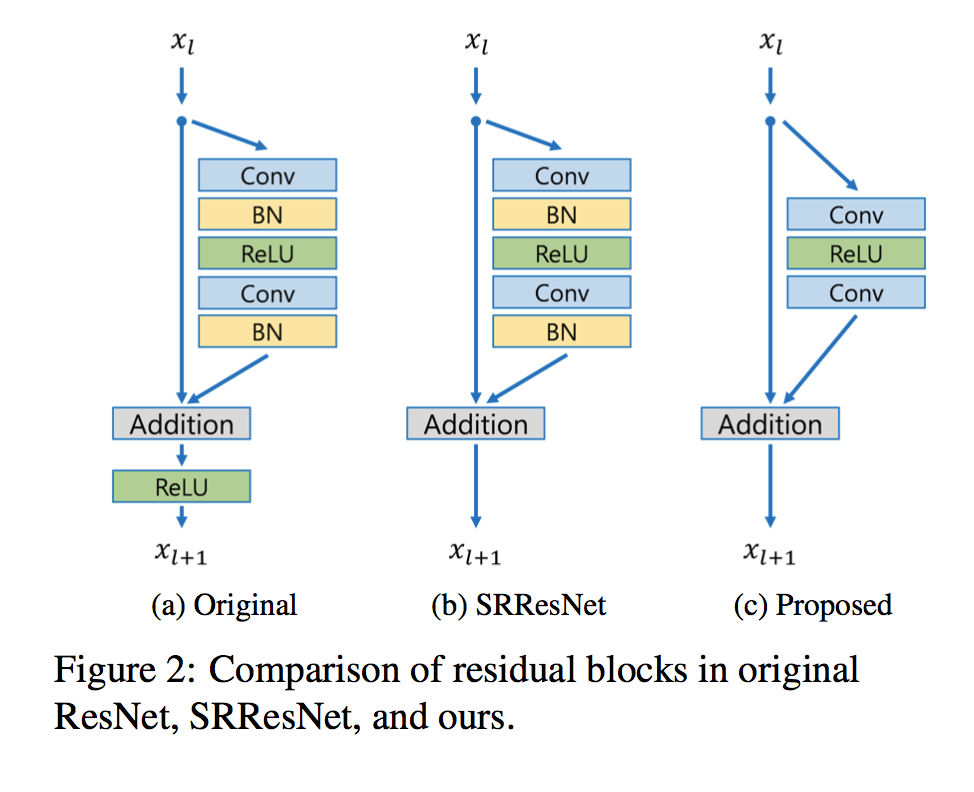import osimport sysimport mathimport torchimport torch.nn as nnimport torch.nn.functional as Fimport numpy as npdef conv(inp, oup, kernel_size, stride=1, dilation=1, groups=1, bias=True):    padding = ((kernel_size -1) * dilation + 1) // 2    return nn.Sequential(        nn.Conv2d(inp, oup, kernel_size, stride=stride, padding=padding, dilation=dilation, groups=groups, bias=bias),    )class ResBlock(nn.Module):    def __init__(self, inp, kernel_size=3, bias=True, bn=False, act=nn.ReLU(True), res_scale=1):        super(ResBlock, self).__init__()        modules = []        for i in range(2):            modules.append(conv(inp, inp, kernel_size, bias=bias))            if bn:                 modules.append(nn.BatchNorm2d(inp))            if i == 0:                 modules.append(act)        self.body = nn.Sequential(*modules)        self.res_scale = res_scale    def forward(self, x):        res = self.body(x).mul(self.res_scale)        res += x        return resresBlock=ResBlock(64)resBlock
Out: ResBlock(  (body): Sequential(    (0): Sequential(      (0): Conv2d(64, 64, kernel_size=(3, 3), stride=(1, 1), padding=(1, 1))    )    (1): ReLU(inplace)    (2): Sequential(      (0): Conv2d(64, 64, kernel_size=(3, 3), stride=(1, 1), padding=(1, 1))    )  ))

## 4、上采样

（1）Interpolation, 比如SRCNN就用简单的三次样条插值进行初步的上采样，然后进行学习非线性映射 。
(2)deconvolution

（3）亚像素卷积层(sub-pixel convolutional layer)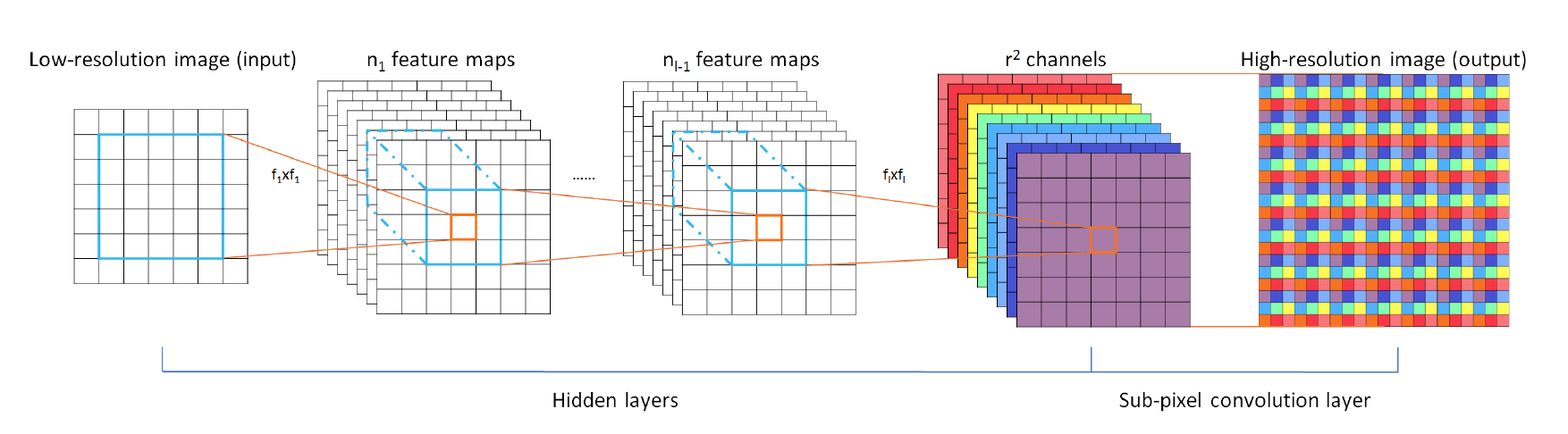class Upsampler(nn.Sequential):    def __init__(self, scale, inp, bn=False, act=False, bias=True, choice=0):        modules = []        if choice == 0: #subpixel           if (scale & (scale - 1)) == 0:    # Is scale = 2^n?               for _ in range(int(math.log(scale, 2))):                   modules.append(conv(inp, 4 * inp, 3, bias=bias))                   modules.append(nn.PixelShuffle(2))                   if bn:                       modules.append(nn.BatchNorm2d(inp))                   if act:                       modules.append(act())           elif scale == 3:               modules.append(conv(inp, 9 * inp, 3, bias=bias))               modules.append(nn.PixelShuffle(3))               if bn:                    modules.append(nn.BatchNorm2d(inp))               if act:                    modules.append(act())           else:               raise NotImplementedError        elif choice == 1: #decov反卷积           modules.append(nn.ConvTranspose2d(inp, inp, scale, stride=scale))        else: #bilinear  #线性插值上采样           modules.append(nn.Upsample(mode='bilinear', scale_factor=scale, align_corners=True))        super(Upsampler, self).__init__(*modules)# tailscale=2inp=64output_channel=3if scale > 1:    tail = nn.Sequential( *[ Upsampler(scale, inp, act = False, choice = 0), conv(inp, 3, output_channel) ] )else:    tail = nn.Sequential( *[ conv(inp, 3, output_channel) ] )tail     
Out: Sequential(  (0): Upsampler(    (0): Sequential(      (0): Conv2d(64, 256, kernel_size=(3, 3), stride=(1, 1), padding=(1, 1))    )    (1): PixelShuffle(upscale_factor=2)  )  (1): Sequential(    (0): Conv2d(64, 3, kernel_size=(3, 3), stride=(1, 1), padding=(1, 1))  ))

## 5、网络架构：

(1)、单尺度SR网络（EDSR）的架构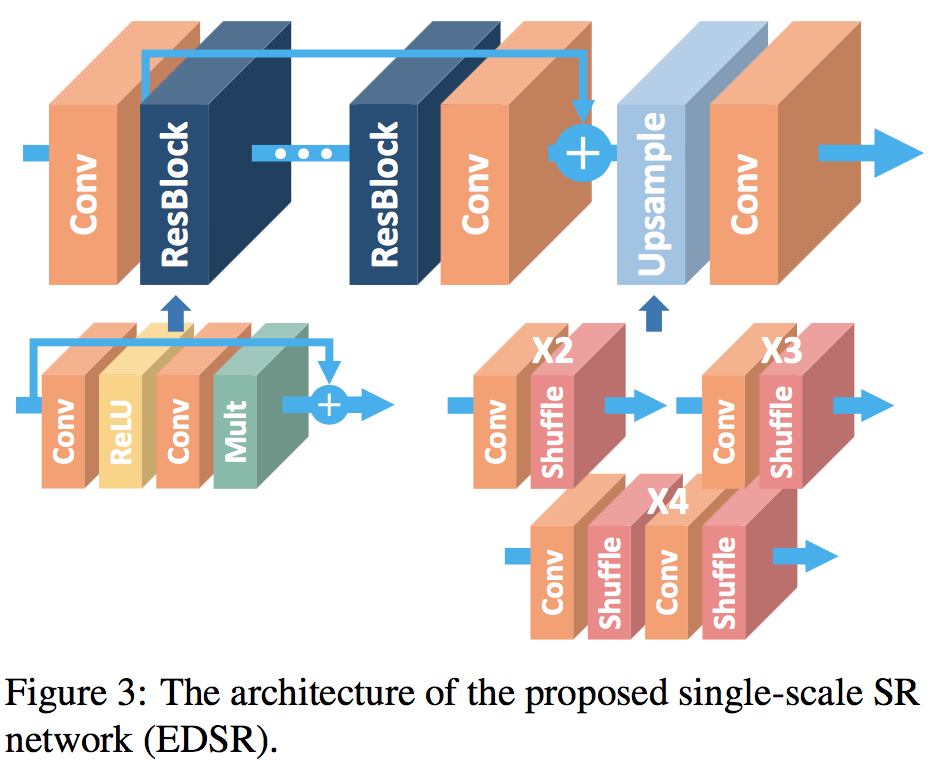(2)、多尺度SR网络（MDSR）的架构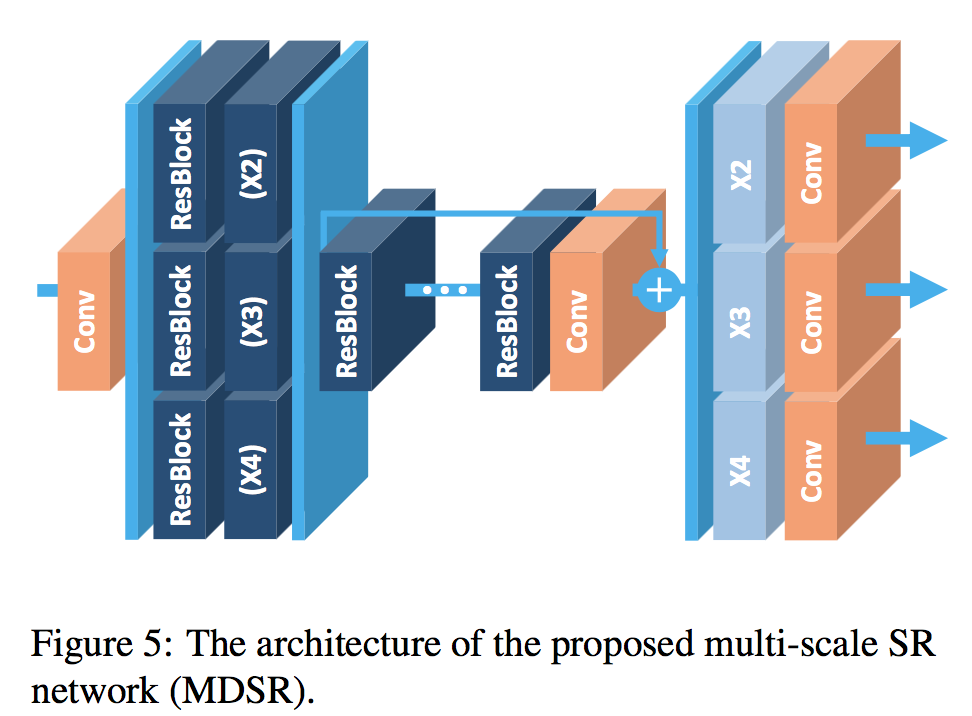## 6、在NTIRE2017超分辨率挑战赛的结果。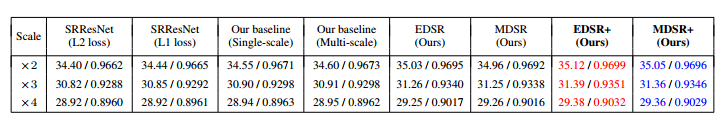## 7、补充：

(1)、PSNR，峰值信噪比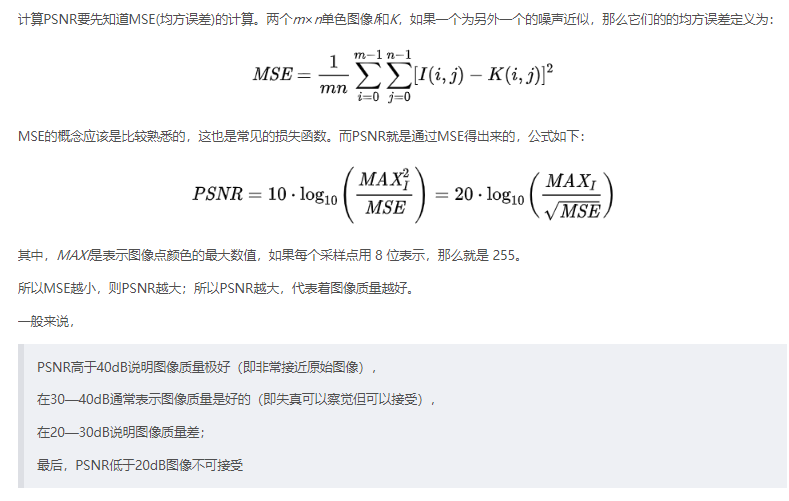(2)、SSIM(structural similarity index)，结构相似性，是一种衡量两幅图像相似度的指标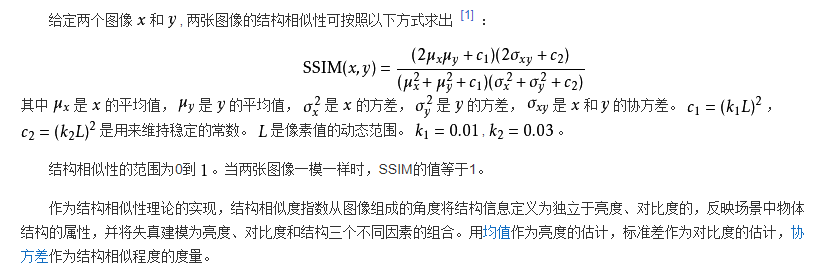• 私有
• 公开
• 删除